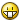Recommended Posts

yello, again... when i use GUIRead... it will only read one number... like so:

GUICtrlSetData (\$edit_23, "= ", 1)

if you used that in a GUI, it only reads 1 number even if "1+2" is entered, it returns "1", just 1, not 1+2... help please?

also, the \$edit_23 is an edit control, maybe it has something to do with that/?

thanks.FootbaG
Share on other sites

2. You can't evaluate a math expression using Number().

There's still no function that does that.

Share on other sites

well that sucks... this is how i found auto-it, i was making a batch file calculator... like the stupid way:

echo off
title Calculator
cls
:start
echo.
echo.
echo   1     2      3
echo.
echo   4     5      6
echo.
echo   7     8      9
echo.
echo                0
echo   +  -
echo.
echo             e
echo.
set choice:
set /p choice= Just use this like a normal Calculator...NOTE: e= Equals
if not '%choice%'=='' set choice=%choice:~0,1%
if '%choice%'=='0+0e' echo 0
if '%choice%'=='1+0e' echo 1
if '%choice%'=='0+1e' echo 1
if '%choice%'=='2+0e' echo 2
if '%choice%'=='0+2e' echo 2
if '%choice%'=='1+2e' echo 3
if '%choice%'=='2+1e' echo 3
if '%choice%'=='3+0e' echo 3
if '%choice%'=='4+0e' echo 4
if '%choice%'=='3+1e' echo 4
if '%choice%'=='1+3e' echo 4
if '%choice%'=='2+2e' echo 4
if '%choice%'=='2+3e' echo 5
if '%choice%'=='3+2e' echo 5
if '%choice%'=='4+1e' echo 5
if '%choice%'=='1+4e' echo 5
if '%choice%'=='5+0e' echo 5
if '%choice%'=='5+1e' echo 6
if '%choice%'=='1+5e' echo 6
if '%choice%'=='4+2e' echo 6
if '%choice%'=='2+4e' echo 6
if '%choice%'=='3+3e' echo 6
if '%choice%'=='6+0e6' echo 6
if '%choice%'=='0+6e6' echo 6
if '%choice%'=='6+1e7' echo 7
if '%choice%'=='1+6e7' echo 7
if '%choice%'=='5+2e7' echo 7
if '%choice%'=='2+5e7' echo 7
if '%choice%'=='4+3e7' echo 7
if '%choice%'=='3+4e7' echo 7
if '%choice%'=='7+0e7' echo 7
if '%choice%'=='0+7e7' echo 7
if '%choice%'=='7+1e8' echo 8
if '%choice%'=='1+7e8' echo 8
if '%choice%'=='5+3e8' echo 8
if '%choice%'=='3+5e8' echo 8
if '%choice%'=='4+4e8' echo 8
if '%choice%'=='6+2e8' echo 8
if '%choice%'=='2+6e8' echo 8
if '%choice%'=='8+0e8' echo 8
if '%choice%'=='0+8e8' echo 8
if '%choice%'=='9+0e9' echo 9
if '%choice%'=='0+9e9' echo 9
if '%choice%'=='8+1e9' echo 9
if '%choice%'=='1+8e9' echo 9
if '%choice%'=='7+2e9' echo 9
if '%choice%'=='7+2e9' echo 9
if '%choice%'=='6+3e9' echo 9
if '%choice%'=='3+6e9' echo 9
if '%choice%'=='5+4e9' echo 9
if '%choice%'=='4+5e9' echo 9
if '%choice%'=='10+0e10' echo 10
if '%choice%'=='0+10e10' echo 10
if '%choice%'=='9+1e10' echo 10
if '%choice%'=='1+9e10' echo 10
if '%choice%'=='8+2e10' echo 10
if '%choice%'=='2+8e10' echo 10
if '%choice%'=='7+3e10' echo 10
if '%choice%'=='3+7e10' echo 10
if '%choice%'=='4+6e10' echo 10
if '%choice%'=='6+4e10' echo 10
if '%choice%'=='5+5e10' echo 10
:0+0e0
goto start
:1+0e1
goto start
:0+1e1
goto start
:2+0e2
goto start
:0+2e2
goto start
:1+2e3
goto start
:2+1e3
goto start
:3+0e3
goto start
:4+0e4
goto start
:3+1e4
goto start
:1+3e4
goto start
:2+2e4
goto start
:2+3e5
goto start
:3+2e5
goto start
:4+1e5
goto start
:1+4e5
goto start
:5+0e5
goto start
:5+1e6
goto start
:1+5e6
goto start
:4+2e6
goto start
:2+4e6
goto start
:3+3e6
goto start
:6+0e6
goto start
:0+6e6
goto start
:6+1e7
goto start
:1+6e7
goto start
:5+2e7
goto start
:2+5e7
goto start
:4+3e7
goto start
:3+4e7
goto start
:7+0e7
goto start
:0+7e7
goto start
:7+1e8
goto start
:1+7e8
goto start
:5+3e8
goto start
:3+5e8
goto start
:4+4e8
goto start
:6+2e8
goto start
:2+6e8
goto start
:8+0e8
goto start
:0+8e8
goto start
:9+0e9
goto start
:0+9e9
goto start
:8+1e9
goto start
:1+8e9
goto start
:7+2e9
goto start
:7+2e9
goto start
:6+3e9
goto start
:3+6e9
goto start
:5+4e9
goto start
:4+5e9
goto start
:10+0e10
goto start
:0+10e10
goto start
:9+1e10
goto start
:1+9e10
goto start
:8+2e10
goto start
:2+8e10
goto start
:7+3e10
goto start
:3+7e10
goto start
:4+6e10
goto start
:6+4e10
goto start
:5+5e10
goto start

it still doesn't work, i was in the middle of it(i know how to make it work) and then i posted for help on a forum and someguy said WOW! why don't you go get autoit and he gave me the link and i was like right... so then i figured out how to fix the batch file calculator, and i didn't, so i went and downloaded autoit, then i got so caught up in how cool it was and forgot about the calculator! until one day i found out that you could just do this for the batch file calculator:

well, i lost it but that's not the point

so yea, idk, i just had to get that outta my system =)

FootbaG
Share on other sites

ah ha, found this little script some person gave me... a long while ago, like a year...

title Simple calculator
@echo off
:calculate
cls
echo enter a math problem
set /p calc="Problem: "
cls
echo %calc% =
echo.
echo.
echo.
echo to abort, type ctrl+break.
echo otherwise,
pause
goto calculate

thats batchfiles of course, so maybe i could send that the problem and then i could retrieve it...

FootbaG

Create an account

Register a new account